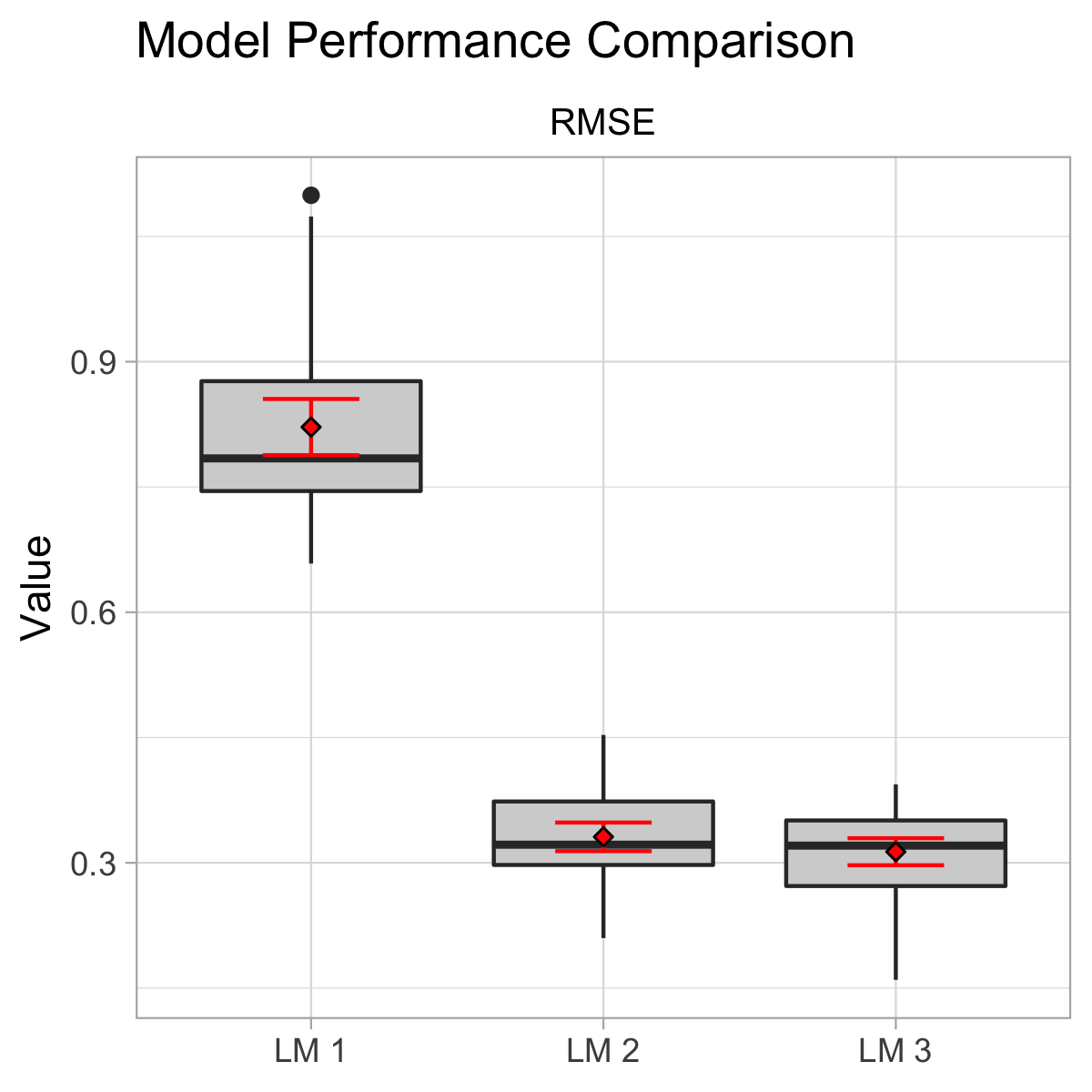Musings about data science in Python and R.
Proceed with caution.

# Recent Posts

### Exploring Leverage in Multivariable Linear Regression

In the context of multivariable linear regression, leverage is a distance measure that shows how far an observation is from the center of the multivariate predictor space. Observations with high leverage values would have the potential to influence the regression model highly while observations with low leverage values would not. Additionally, leverage can be used to determine if a new observation is close to the predictor space of the observations used to create the model in order to avoid extrapolation.

### Supply Source & Route Selection with Linear Programming

Businesses face various types of problems that require making optimal decisions in order to achieve a certain objective. One such problem is the transportation / assignment problem I came across when I was reading the excellent “Spreadsheet Modeling and Decision Analysis” book by Cliff T. Ragsdale. I found this type of problem interesting because the concept can be generalized to many areas of the business. In this post, I will discuss a transportation / assignment problem scenario where we need to select the optimal supply sources & routes that would meet as much of the demand as possible while minimizing the cost of shipping.

# Projects#### MLtoolkit

An R package to help with machine learning & feature engineering tasks.#### Word Predictor

An app that takes an input statement and predicts the next word.#### Predicting Exercise Manner

Predicting exercise manner from accelerometer data.